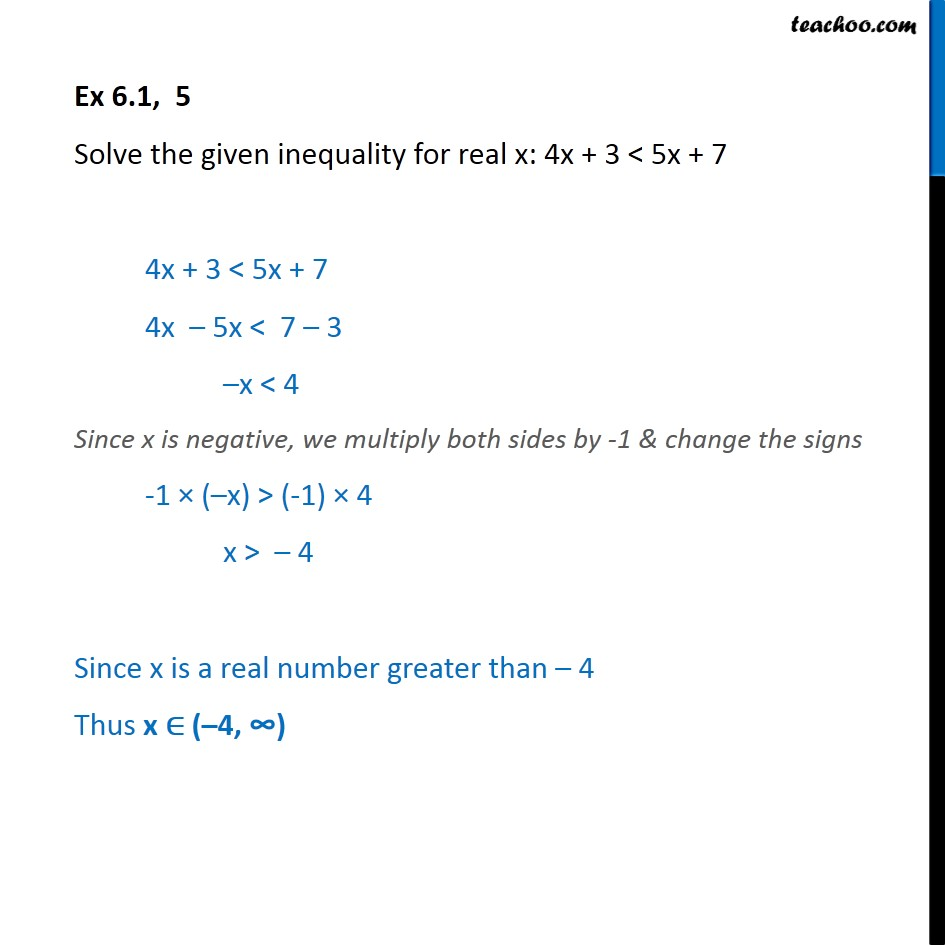Ex 6.1

Chapter 6 Class 11 Linear Inequalities
Serial order wiseGet live Maths 1-on-1 Classs - Class 6 to 12

### Transcript

Ex 6.1, 5 Solve the given inequality for real x: 4x + 3 < 5x + 7 4x + 3 < 5x + 7 4x 5x < 7 3 x < 4 Since x is negative, we multiply both sides by -1 & change the signs -1 ( x) > (-1) 4 x > 4 Since x is a real number greater than 4 Thus x ( 4, )